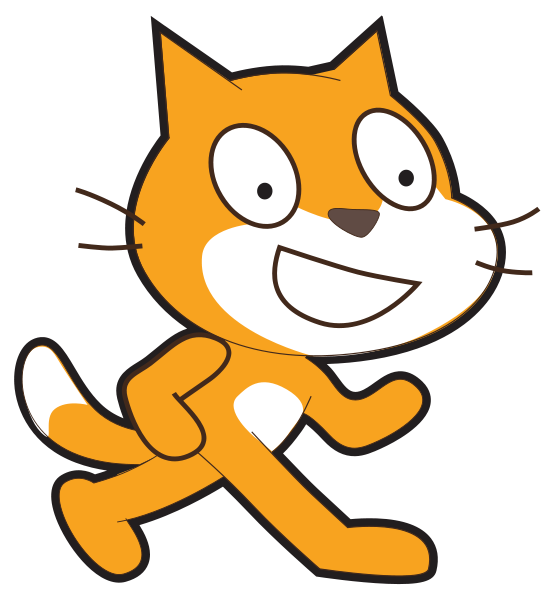# 12 digit product codes: Calculate the last digit (entered one digit at a time)

Learning outcomesStudents will be able to:

## Requirement:

Write a program that asks the user to enter the first 11 digits of a 12 digit product code, one at a time, and works out the last digit as the output.

## Testing examples:

Your program should display the outputs shown in this table for the given inputs provided:

Input Output
6
7
1
8
6
0
0
1
3
6
2
The last digit is: 4
8
3
8
3
1
0
0
2
2
5
2
The last digit is: 4
0
9
3
6
1
6
0
1
6
0
5
The last digit is: 3

## Languages

Scratch#### What it should look like

Click on the green flag, enter the inputs provided in the “testing examples” to see the expected output of your program.

Recommended blocks
when green flag clicked

say (join [The last digit of the product code is ] (last digit))

repeat (5)
end
ask (join (join [Enter digit ] (index)) [ of the product code:]) and wait

ask (join (join [Enter digit ] (index)) [ of the product code:]) and wait

ask (join (join [Enter digit ] (index)) [ of the product code:]) and wait
set [total 1 v] to 

set [total 2 v] to 

set [index v] to 

set [last digit v] to 

change [index v] by (1)

change [total 1 v] by (answer)

change [index v] by (1)

change [total 2 v] by (answer)

change [index v] by (1)

change [total 1 v] by (answer)

set [last digit v] to (((0) - (((total 1) * (3)) + (total 2))) mod (10))
Hints
• You need to add up 1st, 3rd, 5th and so on digits and store the result in a variable called "total 1". Then add up 2nd, 4th, 6th and so on digits and store the result in variable called "total 2". The last digit (check digit) is then calculated by subtracting the sum of "total 1" multiplied by 3, and "total 2" from 0 mod 10.